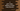# C program to print elements of an array and address of each element### C program to print array elements and address of each element :

In this tutorial, we will learn how to print the address and elements of an integer array. This example will show you how the elements of an array are stored in the memory.

Let’s take a look at the program :

### C program :

``````#include <stdio.h>

int main()
{
//1
int myArray = {1, 2, 3, 4, 5, 6, 7, 8, 9};
int i;

//2
for (i = 0; i < 9; i++)
{
//3
printf("Address for %d is %d\n", myArray[i], &myArray[i]);
}
}``````

### Explanation :

The commented numbers in the above program denote the step number below :

1. Create one integer array myArray with some integer values. We will print these numbers and memory address where it is stored. Integer i is used in the loop below.
2. Run one for loop to read all numbers from the array. Print each number and its address. For printing the address, we are using &myArray[i] for position i.

### Output :

``````Address for 1 is 1489689280